# Render LaTeX in Google Docs

This is for all the students out there!

It turns out that a Google image search for the word “latex” returns many not-safe-for-work images. That’s not the sort of “latex” I’m talking about!

What I’m talking about here is the famous typesetting system, $$\LaTeX$$. If you study anything technical at university (or college for readers in the US who seem to make up the majority of my readers!), you would have come across it.

I use Google Docs a lot. I use it at university, at work, and in my personal life. Being a nerd, I frequently need to write equations in Google Docs. Is there a way to write $$\LaTeX$$ equations in Google Docs?

Yes, there most certainly is!

# How to write and render LaTeX in Google Docs

## Install “Auto-LaTeX Equations”

1. Open up Google Docs and create a new document.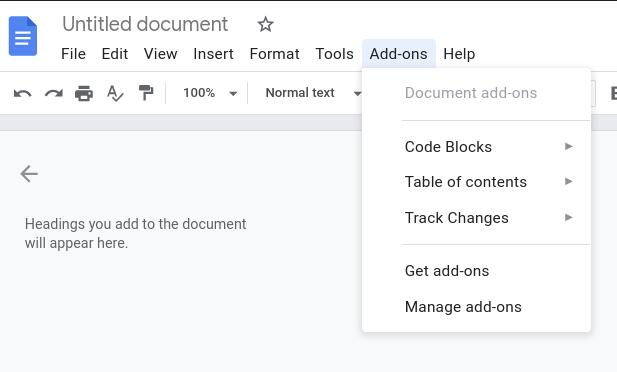1. Search for the word “latex”. The first result should be “Auto-LaTeX Equations. Install it!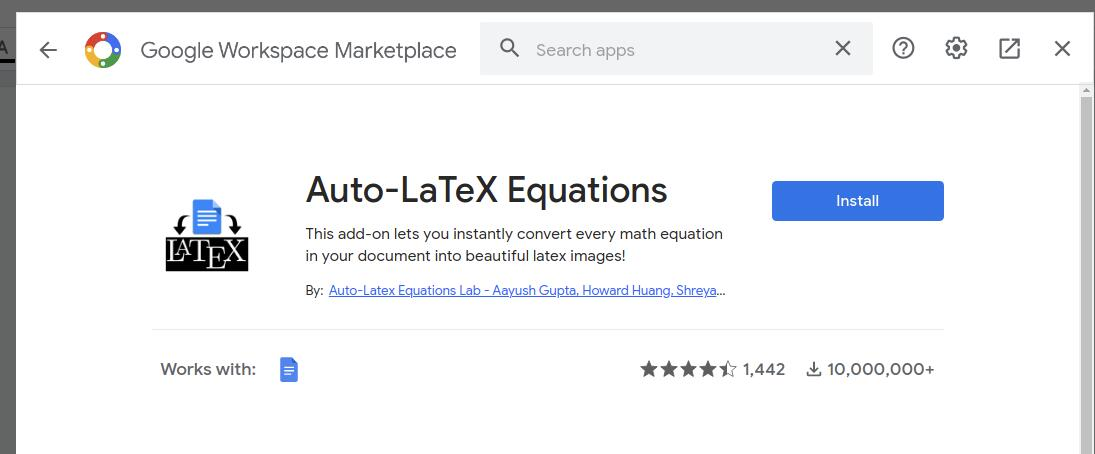1. Once installed, go back to “Add-ons” -> “Auto-LaTeX Equations” -> “Start”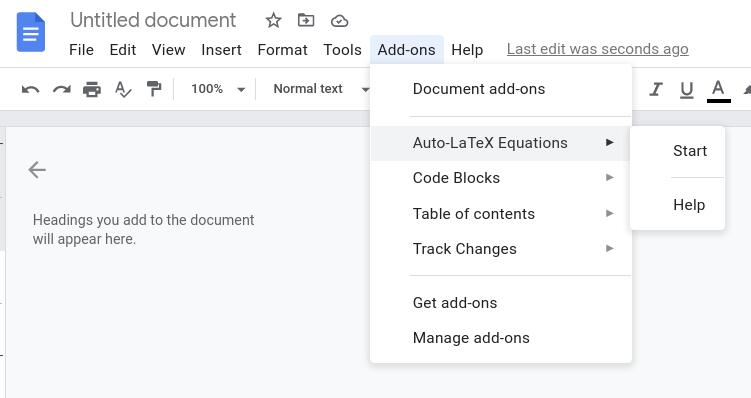The Auto-LaTeX Equations toolbar should appear on the right-hand side of the screen.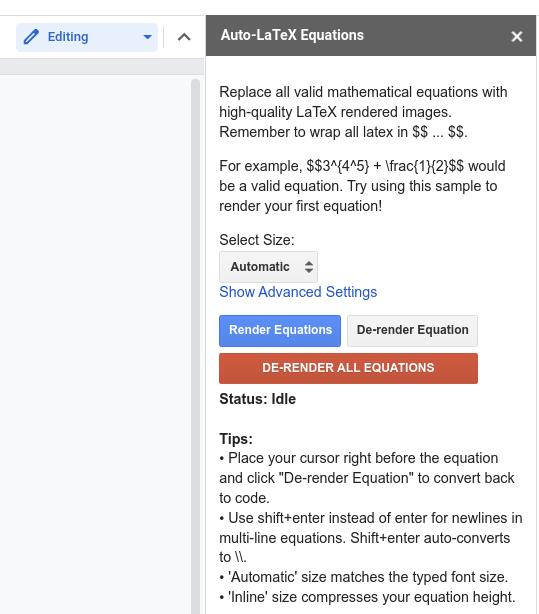Noice.

## Writing single-line equations

Just wrap what you want to render into Latex in double dollar signs.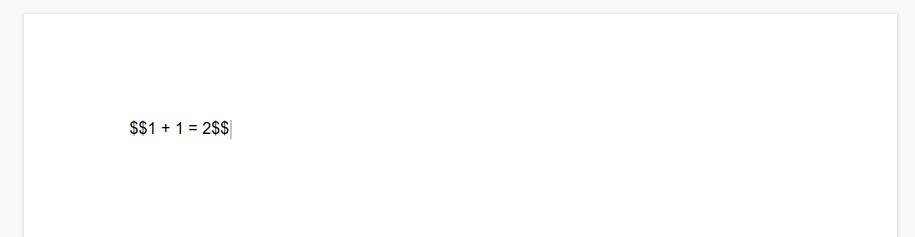Then click on “Render Equations”.After a little while, you should see something like this!Nooooooice.

## Writing multi-line equations

1. Start with two dollar signs, just like with single-line equations. Press shift + enter.
2. Type the LaTeX for your first equation. Press shift + enter.
3. Type the LaTeX for your second equation. Press shift + enter.
4. Type the LaTex for your $n$th equation. Press shift + enter.
5. Type two dollar signs.
6. Press “Render Equations”

Something like this: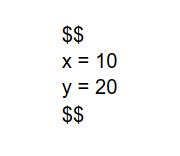will turn into this: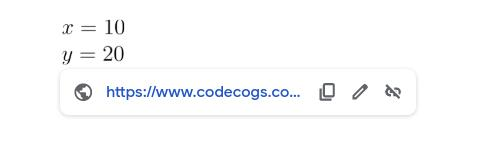Noooooooooooooooice.

# How to write some mathematical things using LaTeX

Here’s a brain dump of things I commonly use.

## Fractions

\frac{1}{2} gives you $$\frac{1}{2}$$

## Less than, greater than, less than or equal to, greater than or equal to

< gives you $$<$$
> gives you $$>$$
\leq gives you $$\leq$$
\geq gives you $$\geq$$

## Exponents and subscripts

x^i gives you $$x^i$$
x^{2n + 1} gives you $$x^{2n + 1}$$
x_{i} gives you $$x_{i}$$
x_{2n + 1} gives you $$x_{2n + 1}$$

## Approximately

\approx gives you $$\approx$$

## Equivalence

\equiv gives you $$\equiv$$

## Sums and products

\sum\limits_{i=1}^{n} x_i gives you $$\sum\limits_{i=1}^{n} x_i$$
\prod\limits_{i=1}^{n} x_i gives you $$\prod\limits_{i=1}^{n} x_i$$

## Partial derivatives

\frac{\partial}{\partial x} x^2 + 3y gives you $$\frac{\partial}{\partial x} x^2 + 3y$$

\nabla f gives you $$\nabla f$$

## That dot from one of the ways to depict a dot product

x \cdot y gives you $$x \cdot y$$

## Big parentheses and brackets

7\left(\frac{x + y}{2}\right) gives you $$7\left(\frac{x + y}{2}\right)$$

z\left[x^2 + 7y\right] gives you $$z\left[x^2 + 7y\right]$$

n\text{th} gives you $$n\text{th}$$

## Arrows like “implies”

\to and \rightarrow give you $$\to$$

\leftarrow give you $$\leftarrow$$

\implies gives you $$\implies$$

## Greek alphabet

They follow a pattern where the lower case variant of the Greek letter begins with a lower case letter. The upper case variant of the same Greek letter begins with an upper case letter. Here are some examples:

\pi and \Pi give you $$\pi$$ and $$\Pi$$
\phi and \Phi give you $$\phi$$ and $$\Phi$$
\theta and \Theta give you $$\theta$$ and $$\Theta$$

## Ellipses

\dots gives you $$\dots$$

## Proper subsets and subsets

\subset gives you $$\subset$$
\subseteq gives you $$\subseteq$$

## Union, intersection

\cup gives you $$\cup$$
\cap gives you $$\cap$$

## Aligning equations

Aligning multi-line equations around equal signs can be done by wrapping it in the align environment. Here’s an example:

\begin{align*}
x &= 20y + z \\
z &= \frac{x}{y}
\end{align*}


# Conclusion

Nooooooooooooooooooooooooooooooooooooooooooooooooooooooooice.

No conclusion, really.

I hope you keep on learning!

Justin

Categories:

Updated: